## Jordan's Lemma

Jordan's lemma shows the value of the Integral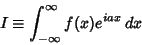(1)

along the Real Axis is 0 for nice'' functions which satisfy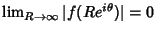. This is established using a Contour Integral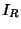which satisfies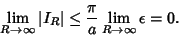(2)

To derive the lemma, write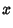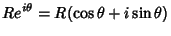(3)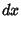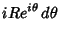(4)

and define the Contour Integral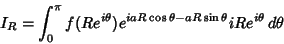(5)

Then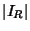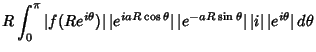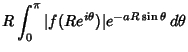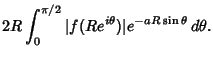(6)

Now, if, choose an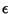such that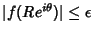, so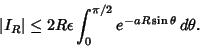(7)

But, for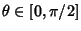,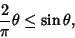(8)

so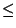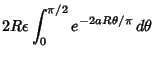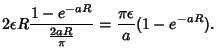(9)

As long as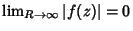, Jordan's lemma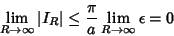(10)

then follows.